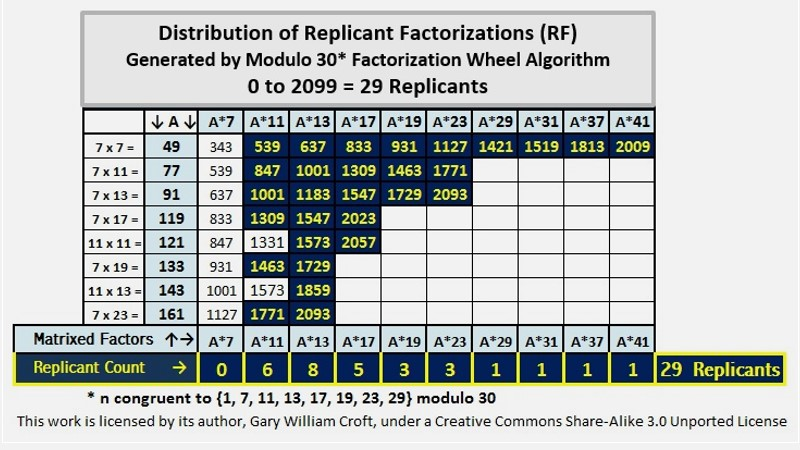# Prime Number Counting Methodology

This work is licensed by its author, Gary William Croft, under a

## Prime Counting π(x)

As we detailed in our discussion of factorization and sieving methods, all composite numbers within the domain of counting numbers not divisible by 2, 3 or 5 can be accounted for algorithmically. We also demonstrated how the number of factorization computations, per se, within specified parameters (from 1 to n) can be given with perfect precision (albeit 'manually' employing spreadsheet formulas). We conjectured, therefore, that upon 'running the numbers' the remaining balance would be primes exclusively (leaving one last step; adding 3 primes to our total to account for 2, 3, and 5, excluded from our domain by definition).

Our logic proved sound ... so far as it went. As you will learn below, we have created a method that eliminates the need for a spreadsheet calculation of factorization dyads from zero to n. What we didn't anticipate was how difficult it is to formulate (rather than mechanically determine) the parsing of equivalent factorizations, i.e., those with identical products but dissimilar factor dyads. For example, of the 105 modulo 30 wheel factorizations that identify (index) all composite numbers in the 0 to 1000 range, we found 5 replicants, namely:

♦  539 =   7 x 77 = 11 x 49
♦  637 =   7 x 91 = 13 x 49
♦  833 = 7 x 119 = 17 x 49
♦  847 = 7 x 121 = 11 x 77
♦  931 = 7 x 133 = 19 x 49

We get a clearer understanding of the sequencing of our five 'replicants' when we express them as prime factorizations:

♦  72 x 11 = 539
♦  72 x 13 = 637
♦  72 x 17 = 833
♦  72 x 19 = 931 (stop: as 72 x 23 = 1127, which is > 997)
♦  112 x 7 = 847 (stop: as 112 x 11 = 1331, which is > 997)
(and, of course 132 x 7 = 1183 also exceeds 997, the limit of our defined range ... STOP! as does 7 x 11 x 13 = 1001)

For now we can state that, although Replicant Factorizations (RF's) can be easily explained, quantifying them formulaically remains a daunting challenge given we're seeking exactitude, not "error approximation."

That being said, using our method as it currently stands (i.e., wanting a better RF counting method) what follows are steps to calculate the number of primes within three arbitrarily chosen ranges, and a fourth example chosen literally at random (throwing a dart at a printed page containing several hundred prime numbers):

[Note: π(x) is the standard notation indicating the count of prime numbers between 1 and x that notoriously misappropriates the pi symbol.]
π(10,000) = 1229
π(1,000) = 168
π(892) = 1000
π(2099) = 317

Example 1: π(10,000) = 1229

1. First, calculate the number of natural numbers from 1 to 10,000 not divisible by 2, 3, or 5, i.e., n ≡ {1, 7, 11, 13, 17, 19, 23, 29} modulo 30. Since 8 out of every 30 natural numbers {1, 2, 3, ...} fall under this modular set, we multiply the highest number less than 10,000 that is n ≡ {1, 7, 11, 13, 17, 19, 23 or 29} modulo 30 (in this case 9,997) times 8/30, or .2666 (8/30 being the ratio of numbers not divisible by 2, 3, or 5 to natural numbers). And thus, 9,997 x 8/30 = ⌊2665⌋ (floor brackets signify whole number part taken). This is our starting point. The steps that follow will identify the number of composite numbers to subtract by a counter-intuitive process of elimination until we've reduced 2665 down to the point where only prime numbers remain.
2. Given that the square root of 9,997 is 99.98, we know that we need to perform chord factorizations from 7chord to 97chord to identify composite numbers in our range less than or equal to 9,997 (reference "The 8-Chord Progression," above). Doing so will index all composite numbers in this range (as distinct from numbers divisible by 2, 3 and 5, already removed in the previous step).
3. We then count the number of composite generating calculations required to complete step 2. This gives us 1847 (keeping in mind that this number includes 408 RF's, the counting of which is illustrated below). (To open an Excel spreadsheet showing in detail how this number is derived, click here.) (And below we offer a formulaic alternative to this manual counting routine.).
4. Next we sum and subtract Redundant Factorizations from the total number of factorization calculations generated in step 3 above. In our designated range (1 thru 9,997), we find that there are 408 such duplications. Subtracting these from the total number of factorizations (1847 minus 408) gives us 1439. To open an Excel spreadsheet listing the duplicates and their sums, click here.
5. Next, we subtract the result in step 4 (1439) from the result in step 1 (2665), i.e., 2665 minus 1439 = 1226.
6. Lastly, we must circle back and add 3 to our total to account for primes 2, 3 and 5, which by definition were excluded from our factorization domain. And, voila!, this brings us to 1226 plus 3 = 1229, the exact number of primes among the first 10,000 natural numbers.

Earlier we alluded to a novel method we've invented to eliminate the need for manual counting of the algorithmically generated factorizations described in Step 3. This simplified formulation, which could form the basis of a function, is magical in that it incrementally trues the delta difference between the actual and calculated sums in perfect stair-step fashion: 0, 1, 2, 3, ... 24, additively summing to 300. This 0, 1, 2, 3, ... n cumulative summation in the form n(n+1)/2 occurs with electrifying regularity no matter the highest n of a defined range, as the examples below demonstrate. [Note: Below (directly following the graphic illustrating this example) we describe a more direct alternative approach that eliminates the need for this n(n+1)/2 delta truing.]

Before diving into our example, however, its instructive to expose the powerful role played by the ratio of numbers not divisible by 2, 3, or 5 to natural numbers 1, 2, 3, ... or 8/30 = .2666 ... and its inverse, 30/8 = 3.75, demonstrated by the equations that follow.

For starters, we offer two approaches to calculating the index numbers, n 1, 2, 3, ..., of numbers not divisible by 2, 3, or 5. The first method is expedient, but if you're a purist it has the downside of producing results with fractional parts, namely:

n ≡ {1, 7, 11, 13, 17, 19, 23, 29} modulo 30:
k = n * 8/30 + 1 = natural numbers ⌊1, 2, 3, ...⌋ ⌊whole number part taken⌋
.
For example, 89 * 8/30 + 1 = 24.7333 (or 24, when whole number part taken). And 89 is indeed the 24th member of our domain. By comparison, the 'precise' equation for any n ≡ {29} modulo 30, which includes number 89, is k = (n + 1) * 8/30; and thus (89 + 1) * 8/30 = 24. More about the latter equation, member of an octad, follows.

The second approach is more laborious, but produces precise whole number results. The 8-fold conversion equations, parsed by modulo 30 congruence, are listed in the left-hand column of the table below. The equations in the right-hand column produce cumulative sums from 1 to a given n not divisible by 2, 3, or 5. To demonstrate how these equations interrelate, we follow with an example employing the cumulative sum from 1 to 7919 (the 1000th prime number) in relation to its index number: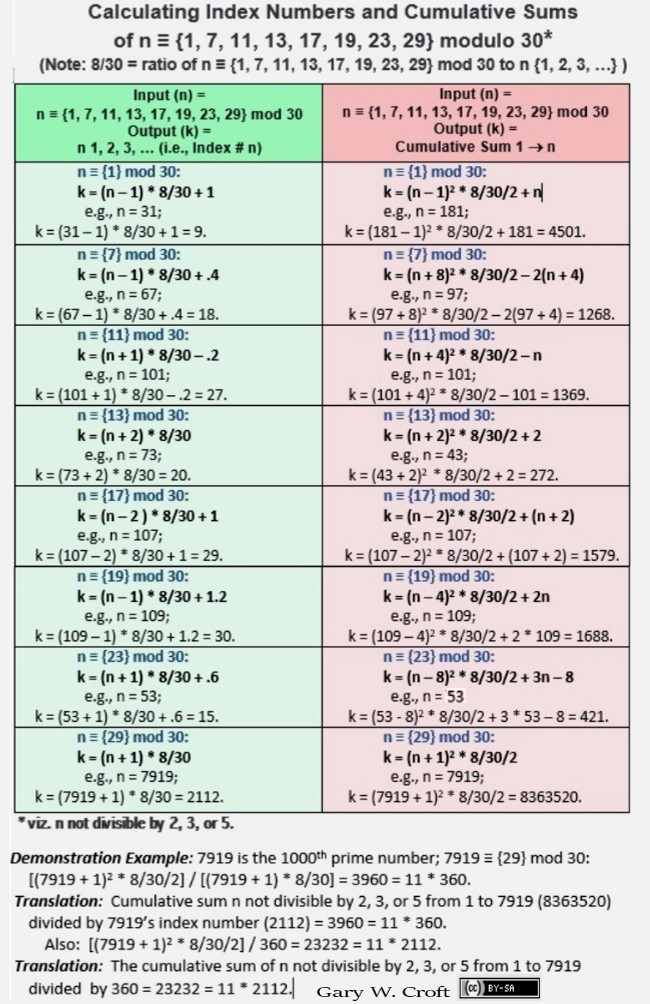Equations to generate the inverse of the above, i.e., input (n) = counting numbers 1, 2, 3, ... and output (k) = n not divisible by 2, 3, or 5, are shown below:

k = n * 30/8 {-2.75, -0.50, -0.25, -2.00, -1,75, -3.50, -3.25, -1.00} {repeat ...}
= n ≡ {1, 7, 11, 13, 17, 19, 23, 29} modulo 30
.

Parsing the inputs by their modulo 8 congruence allows us to input index numbers individually to determine a specific number's output (Note that the 8 negative adjustments per cycle form 4 bilateral pairs summing to 3.75, and 4 * 3.75 = 15.).

n ≡ {1} mod 8: k = n * 30/8 − 2.75 = n ≡  {1}  mod 30
n ≡ {2} mod 8: k = n * 30/8 − 0.50 = n ≡  {7}  mod 30
n ≡ {3} mod 8: k = n * 30/8 − 0.25 = n ≡ {11} mod 30
n ≡ {4} mod 8: k = n * 30/8 − 2.00 = n ≡ {13} mod 30
n ≡ {5} mod 8: k = n * 30/8 − 1.75 = n ≡ {17} mod 30
n ≡ {6} mod 8: k = n * 30/8 − 3.50 = n ≡ {19} mod 30
n ≡ {7} mod 8: k = n * 30/8 − 3.25 = n ≡ {23} mod 30
n ≡ {0} mod 8: k = n * 30/8 − 1.00 = n ≡ {29} mod 30

Here are the first 24 outputs of the equations

k = 1 * 30/8 − 2.75 = 1
k = 2 * 30/8 − 0.50 = 7
k = 3 * 30/8 − 0.25 = 11
k = 4 * 30/8 − 2.00 = 13

k = 5 * 30/8 − 1.75 = 17
k = 6 * 30/8 − 3.50 = 19
k = 7 * 30/8 − 3.25 = 23
k = 8 * 30/8 − 1.00 = 29

k = 9 * 30/8 − 2.75 = 31
k = 10 * 30/8 − 0.50 = 37
k = 11 * 30/8 − 0.25 = 41
k = 12 * 30/8 − 2.00 = 43

k = 13 * 30/8 − 1.75 = 47
k = 14 * 30/8 − 3.50 = 49
k = 15 * 30/8 − 3.25 = 53
k = 16 * 30/8 − 1.00 = 59

k = 17 * 30/8 − 2.75 = 61
k = 18 * 30/8 − 0.50 = 67
k = 19 * 30/8 − 0.25 = 71
k = 20 * 30/8 − 2.00 = 73

k = 21 * 30/8 − 1.75 = 77
k = 22 * 30/8 − 3.50 = 79
k = 23 * 30/8 − 3.25 = 83
k = 24 * 30/8 − 1.00 = 89

Getting back to our example (counting primes from 0 → 10,000), when you subtract the sum of the cumulative corrections (300, in this case) from the total number of calculations (2147 minus 300) you get 1847, perfectly matching the actual count. [Note the taking of whole number parts (Columns C and E) and the downward adjustment (when necessary) of Column C results to n ≡ {1, 7, 11, 13, 17, 19, 23 or 29} modulo 30; the result posted under Column D.]:* Note: Columns C & E: Whole Number Part TakenAbove we noted the discovery of an alternative to the additive factorization sum 'truing' equating to n(n+1)/2, namely: E − (A * 8/30 − 2) = ⌊F⌋ = Actual Number of Factorizations ⌊whole number part taken⌋, as follows:

E −   (A * 8/30 − 2) =   ⌊F⌋
380 −   (7 * 8/30 – 2) = ⌊380⌋
241 − (11 * 8/30 – 2) = ⌊240⌋
205 − (13 * 8/30 – 2) = ⌊203⌋
156 − (17 * 8/30 – 2) = ⌊153⌋
139 − (19 * 8/30 – 2) = ⌊135⌋
115 − (23 * 8/30 – 2) = ⌊110⌋
91 − (29 * 8/30 – 2) =   ⌊78⌋
85 − (31 * 8/30 – 2) =   ⌊85⌋
71 − (37 * 8/30 – 2) =   ⌊63⌋
64 − (41 * 8/30 – 2) =   ⌊55⌋
61 − (43 * 8/30 – 2) =   ⌊51⌋
56 − (47 * 8/30 – 2) =   ⌊45⌋
54 − (49 * 8/30 – 2) =   ⌊42⌋
49 − (53 * 8/30 – 2) =   ⌊36⌋
45 − (59 * 8/30 – 2) =   ⌊31⌋
43 − (61 * 8/30 – 2) =   ⌊28⌋
39 − (67 * 8/30 – 2) =   ⌊23⌋
37 − (71 * 8/30 – 2) =   ⌊20⌋
35 − (73 * 8/30 – 2) =   ⌊17⌋
33 − (77 * 8/30 – 2) =   ⌊14⌋
32 − (79 * 8/30 – 2) =   ⌊12⌋
31 − (83 * 8/30 – 2) =   ⌊10⌋
29 − (89 * 8/30 – 2) =     ⌊7⌋
29 − (91 * 8/30 – 2) =     ⌊6⌋
27 − (97 * 8/30 – 2) =     ⌊3⌋

The Total equals 1847, the exact count of factorizations within this domain between 1 and 10,000 when generated by the modulo 30 algorithms discussed earlier.

Before leaving this example, it's instructive to study the patterns generated by the replicant factorizations within this range. Below is a screen shot from a spreadsheet that color codes the 408 replicant factorizations between 1 and 10,000, revealing their straight-line sequencing, extending both vertically and horizontally. (This is a sample: For the entire spreadsheet, click here: Replicant Factorization Patterns; tab labeled "Factorization Inventory").From the spreadsheet linked above, parsing and tiering the distribution of Replicant Factorizations by their modulo 30 vertical alignment gives us the following counts: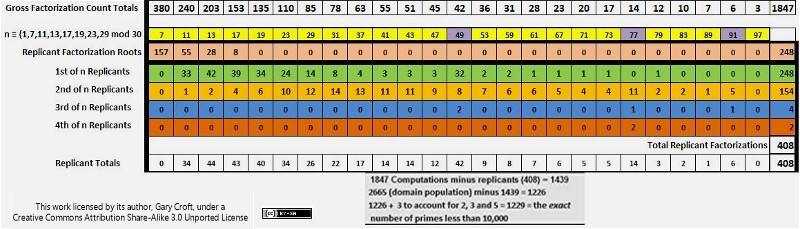Example 2: π(1,000) = 168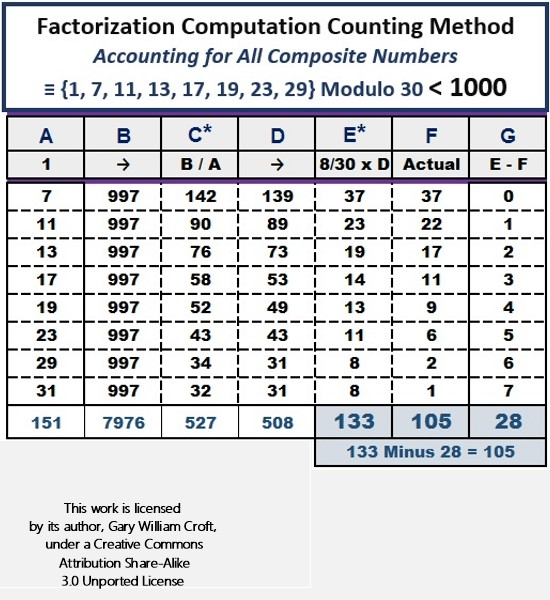* Note: Columns C & E: Whole Number Part Taken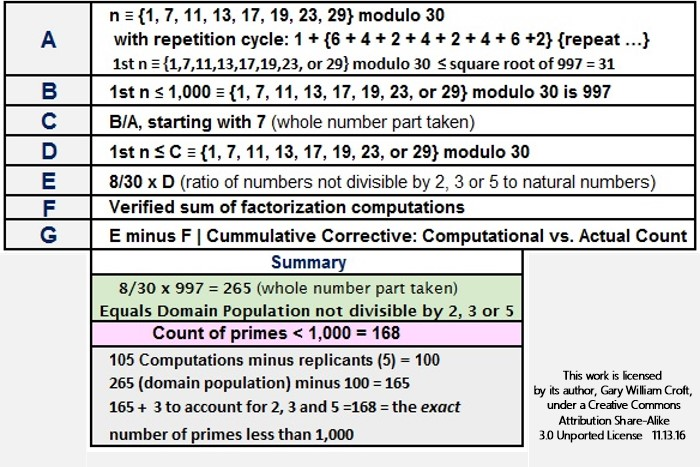Example 3: π(892) = 1000

As we've noted several places on this site, there are exactly 1000 prime numbers between 1 and 892, i.e., between 1 and 7921. Here are the steps that get us to 1000:

1. Calculate population of the modulo 30 defined domain (see step 1 of the 1st example), thus: 7921 x 8/30 = 2112 (taking the whole number part). [Note: given 7921 is congruent to {1} modulo 30, and is therefore a member of our domain, we've pegged it as the highest number in our range.]
2. Take the square root of 7921 (which is 89) to determine the high end of our range of chordal factorizations for counting purposes.
3. Count number of sequenced factorization calculations (7 thru 89): 1396.
4. Count and subtract number of duplicate calculations to produce net number: 1396 minus 281 = 1115.
5. Subract 1115 from the domain population determined in step 1: 2112 minus 1115 = 997.
6. Add 3 to account for primes 2, 3, and 5: 997 plus 3 = 1000, the exact number of primes between 1 and 892!

Consistent with the less than 1000 and less than 10000 factorization counting function, we can take the same approach with 892: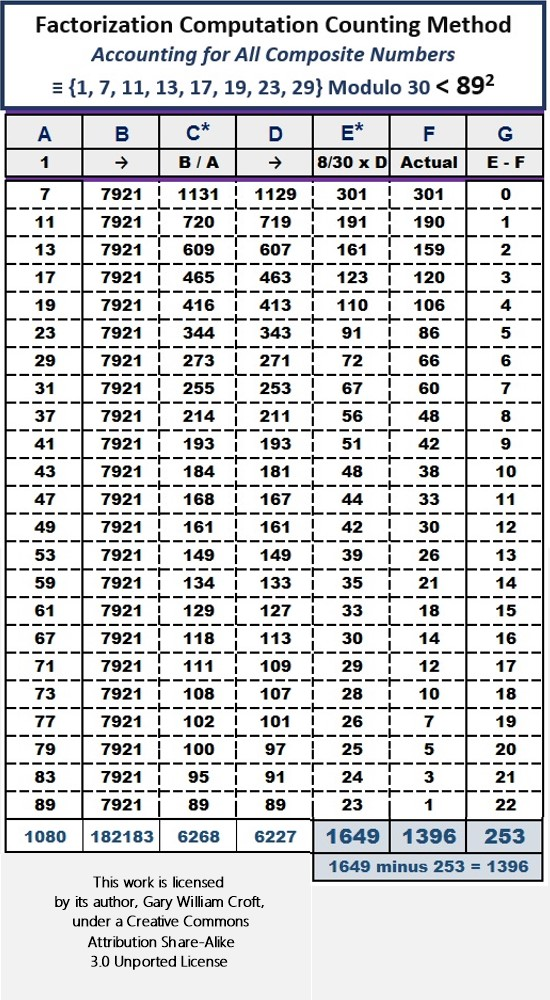* Note: Columns C & E: Whole Number Part TakenHere's a link to an Excel.xlsx spreadsheet with three tabs containing data supporting the counts in the "Primes Less than 89 Squared" Summary, directly above: 1) Actual Factorization Counts; 2) Calculated Counts; 3) Replicant Analysis: 89 Squared and the First 1000 Prime Numbers.

In a related set of experiments, prompted by the fascinating and suggestive properties of number 89 and its square, we've calculated the delta differences between the squares of numbers congruent to 89 modulo 90 that are evenly divisible by 360 and 1000, and have compiled a growing list of examples like those illustrated below (which are truly mind-blowing). Our conjecture is that the 24-fold modulo 90 factorization sequences described on this site periodically synchronize, 'leaving behind' prime number counts evenly divisible by 360 and/or 1000.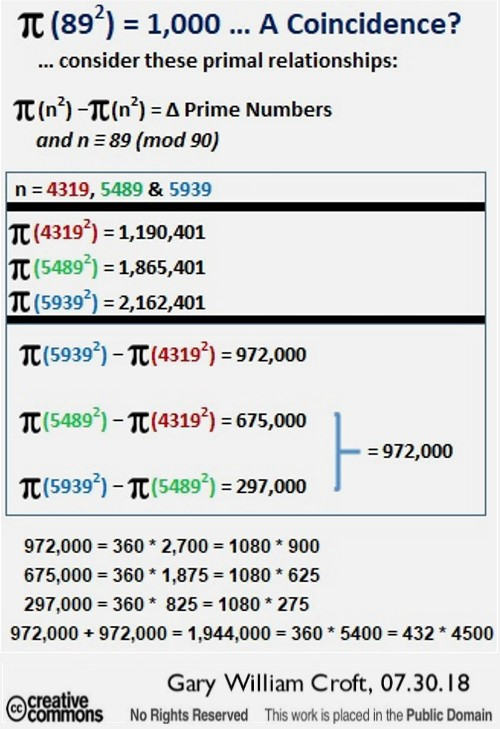And here's a related formulation that begs further study: [Note: In the equation illustrated below, where (89 * 8/30 +1) * (89 +1)/2 = 1113, the whole number part is not taken for (89 * 8/30 +1), unlike in the equation shown for index numbers of n not divisible by 2, 3, or 5, where whole number parts are indeed taken, e.g., 89's index number equates to [89 * 8/30 +1] = , and when you plug this into the equation for the cumulative sum of n congruent to {29} mod 30 you get [89 * 8/30 +1] * (89 +1) / 2 = 1080.]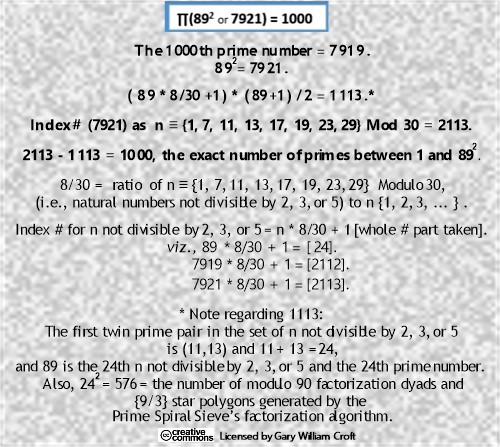Example 4: π(2099) = 317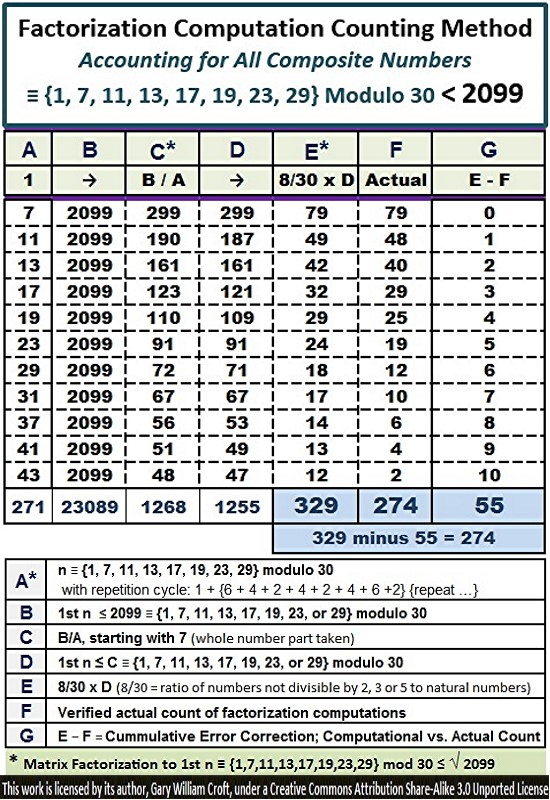* Note: Columns C & E: Whole Number Part Taken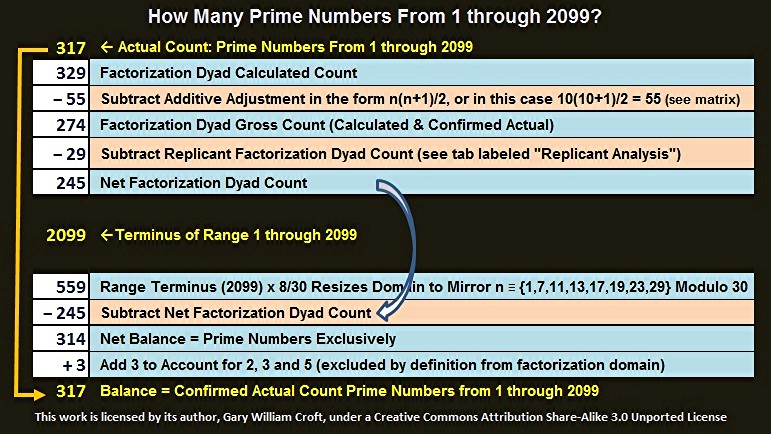Examining the 29 Replicant Factorizations distributed between zero to 2099 unambiguously reveals their genesis. The question remains how to parse them formulaically.